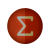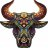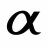# Trouble defining variables based on current symbol

J

#### jpbcx43

##### New member
I have a script that plots price lines based on some calculations, however I am currently defining variables based on inputs.

The goal here is to define variables dynamically based on the current symbol.

Example
If the current symbol is AMD, define Price1 as 50 and Price2 as 20.
If the current symbol is SPX, define Price1 as 3000 and Price2 as 2900.

For some reason, this logic is displaying "Invalid statement: if at 1:1" and "Syntax error: Semicolon expected at 1:1". Can anyone help? I really can't tell what I am missing, or if switch statement would be better.

Code:
``````if GetSymbol() == "SPX" {
Price1 = "2900";
Price2 = "3000";
} else

if GetSymbol() == "AMD" {
Price1 = "50";
Price2 = "20";
} else  {
Double.NaN;
}``````

J

#### jpbcx43

##### New member
I was able to accomplish something in the meantime, however I am not sure this is the most efficient way of defining the variables:

Code:
``````def Price1 = if (GetSymbol() == "AMD") then 50
else if (GetSymbol() == "SPX") then 2900
else if (GetSymbol() == "SPY") then 290
else if (GetSymbol() == "AAPL") then 286
else if (GetSymbol() == "STZ") then 164
else Double.NaN;

def Price2 = if (GetSymbol() == "AMD") then 20
else if (GetSymbol() == "SPX") then 3000
else if (GetSymbol() == "SPY") then 300
else if (GetSymbol() == "AAPL") then 292
else if (GetSymbol() == "STZ") then 168
else Double.NaN;``````

O

#### optiwatch

##### New member
Define the variables above your conditional statement. If you assign a value in an IF statement, you must make sure each ...IF, ELSE IF, ELSE assigns also.

Code:
``````def Price1;
def Price2;
if GetSymbol() == "SPX" {
Price1 = 2900;
Price2 = 3000;
} else if GetSymbol() == "AMD" {
Price1 = 50;
Price2 = 20;
} else  {
Price1 = Double.NaN;
Price2 = Double.NaN;
}``````

Also, the current symbol is the default when you use most Thinkscript functions (just use high, low, close, open, etc)
I prefer to make the numbers fully dynamic:
Code:
``````input percent = 1;
def Price3 = close * (1 - percent/100);
def Price4 = close * (1 + percent/100);``````

J

#### jpbcx43

##### New member
Thank you kindly sir! Much appreciated.Defining the index a symbol is apart of Questions 3Need help with array variables Questions 1Recursive variables Questions 3